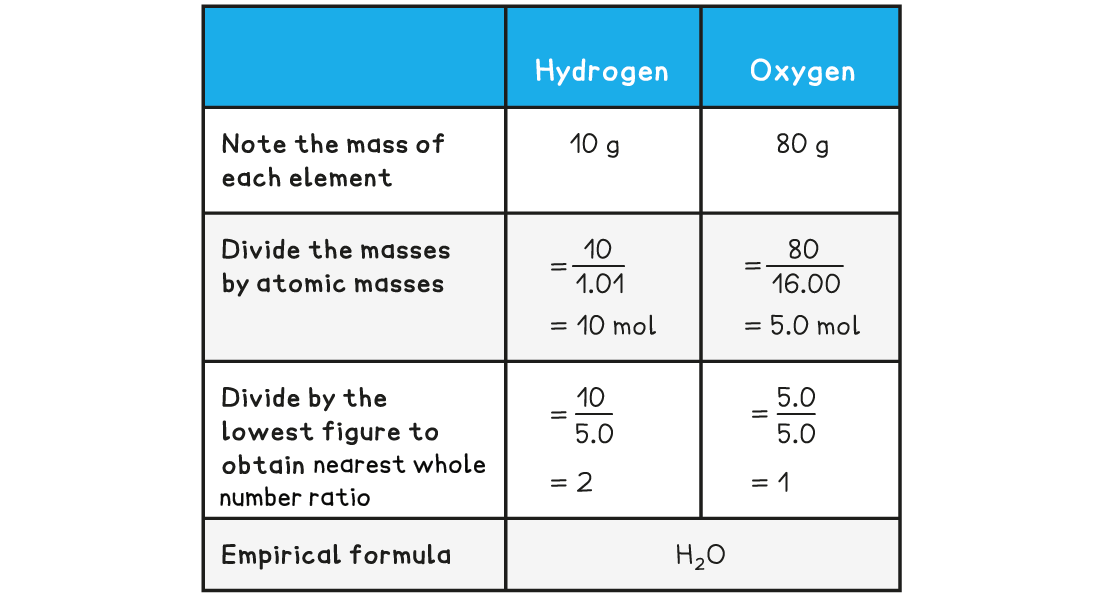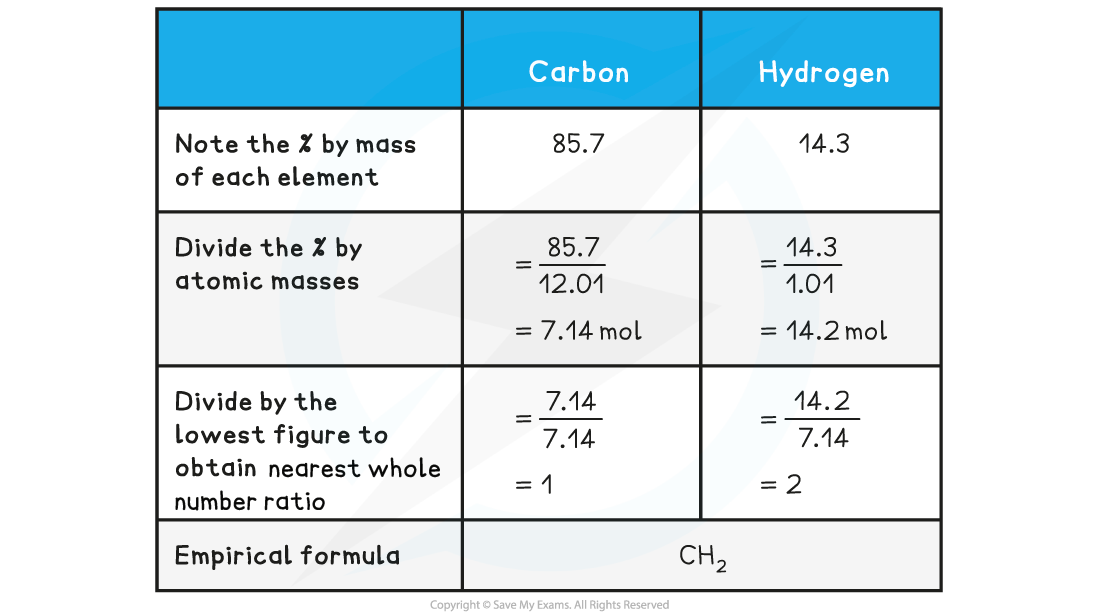# IB DP Chemistry: SL复习笔记1.1.6 Empirical Formulae

### Empirical & Molecular Formulae

• The molecular formula is the formula that shows the number and type of each atom in a molecule
• E.g. the molecular formula of ethanoic acid is C2H4O2
• The empirical formula is the simplest whole number ratio of the atoms of each element present in one molecule or formula unit of the compound
• E.g. the empirical formula of ethanoic acid is CH2O
• Organic molecules often have different empirical and molecular formulae
• The formula of an ionic compound is always an empirical formula

### Empirical Formula Calculations

#### Empirical formula

• The empirical formula is the simplest whole number ratio of the atoms of each element present in one molecule or formula unit of the compound
• It is calculated from a knowledge of the masses of each element in a sample of the compound
• It can also be deduced from data that give the percentage composition by mass of the elements in a compound

#### Worked Example

Determine the empirical formula of a compound that contains 10 g of hydrogen and 80 g of oxygen

Answer:#### Worked Example

Determine the empirical formula of a compound that contains 85.7% carbon and 14.3% hydrogen#### Molecular formula

• The molecular formula gives the actual numbers of each element present in the formula of the compound
• The molecular formula can be found by dividing the relative molecular massby the relative mass of the empirical formula and finding the multiple that links the empirical formula to the molecular formula
• Multiply the empirical formula by this number to find the molecular formula

#### Worked Example

The empirical formula of X is C4H10S and the relative molecular mass of X is 180.42. What is the molecular formula of X? Relative Atomic Mass    Carbon: 12.01    Hydrogen: 1.01    Sulfur: 32.07

Step 1: Calculate the relative mass of empirical formula

Relative empirical mass = (C x 4) + (H x 10) + (S x 1)

Relative empirical mass = (12.01 x 4) + (1.01 x 10) + (32.07 x 1)

Relative formula mass = 90.21

Step 2: Divide relative molecular mass of X by relative mass of empirical formula

The multiple between X and the empirical formula = 180.42/90.21 = 2

Step 3: Multiply the empirical formula by 2

2 x C4H10S    =    C8H20S2

The molecular formula of X is C8H20S2